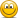YouTubeT3-13: Par yields are swap rates

Nicole Seaman

Director of FRM Operations
Staff member
Subscriber
The par yield is the coupon rate that prices a bond to par. It is also effectively the swap rate.

David's XLS is here: https://trtl.bz/2HPIDMX

Last edited:

danghara

Member
Why are the par yields slightly less than spot rates(for upward sloping curve)?
It seems to be identical!!!
What is the cause of their difference?

Last edited:

ami44

Well-Known Member
Subscriber
I thought this is easy to show, but then I found it surprisingly difficult.

Intuitive explanation:
The par rate is the rate of at which a swap has a value of zero. Each coupon payment is discounted with the spot rate of it‘s payment date. So the par rate is some kind of average of the spot rates, which are lower than the spot rate at maturity (upward sloping). So the par rate is lower than the spot rate at maturity.

Mathematical explanation:
Swap has $$n$$ annual coupon payments and forward and discount curve are the same.
Par rate: $$r_p$$
Spot rates: $$z_i$$
For a spot at par we have

$$1 - e^{-z_n \cdot n} = (e^{r_p} - 1) \sum_{i=1}^n{e^{-z_i \cdot i}}$$
Be aware that I used $$r_p$$ continuously compounded, just so, that spot and par rate have the same compounding.

We know that if the yieldcurve is flat, i.e. all $$z_i$$ are the same, than also the par rate is equal to that rate $$r_p = z_i$$.
This is mentioned by David in this post, but if you are interested, I think the proof is not too complicated.

Solving above equation for the parrate:
$$e^{r_p} - 1 = \frac{1-e^{-z_n \cdot n}}{\sum{e^{-z_i \cdot i}} }$$

Since we know that $$r_p=z_n$$ if $$z_i = z_n$$ for all $$i$$ we can conclude that $$r_p < z_n$$ if the $$z_i$$ are lower than $$z_n$$ (upward slope), because lower $$z_i$$ result in a greater denominator, which than result in a lower par rate.

If someone has an easier explanation, i‘m interested.

danghara

Member
hello ami44
thank you very much for your clean explanationDenisAmbrosov

New Member
Subscriber
Can't understand why the swap rates and the par rates are equal. What are swap rates and why they are not equal to spot rates(why spot rates are a little bit higher than swap rates). Spot rates are the rates observed in the market?
Thanks!

David Harper CFA FRM

David Harper CFA FRM
Staff member
Subscriber
Hi @DenisAmbrosov I moved your question (it's the sort of question that's easy to search, and has been asked many times, for good reasons! We have several discussions on it). See above. Thanks,